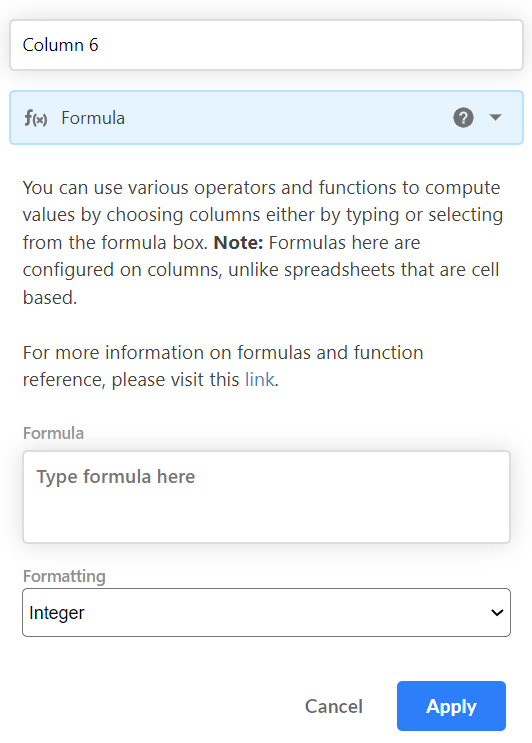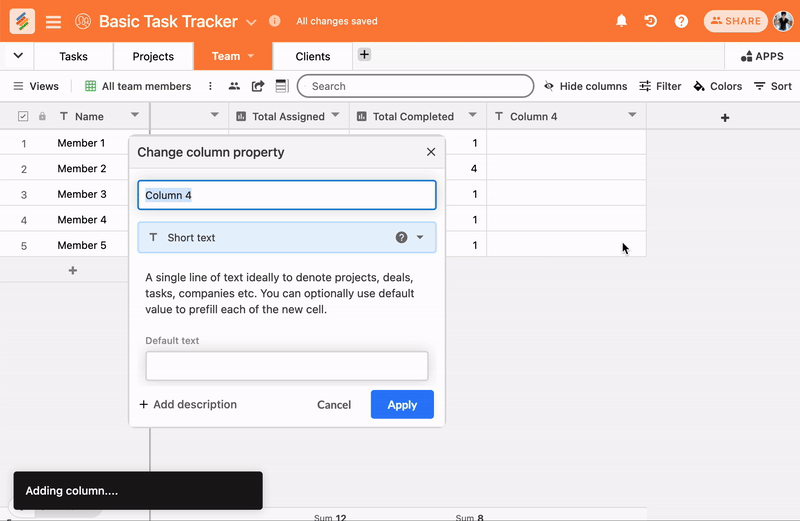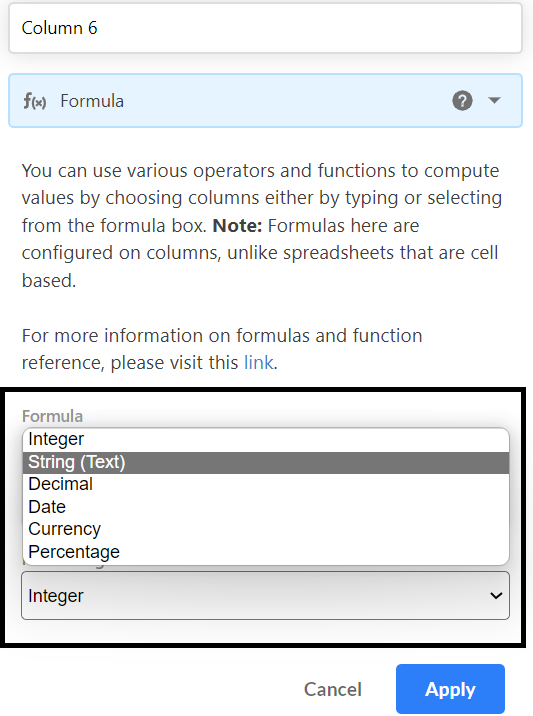Stackby consists of more than 25 columns to add in the table from that one is FORMULA column type. Formulas let you reference other columns in a table and create different functions based on the content of those tables.

1. How to write formula
2. Formatting of formula column type

Creating a formula column type

Add new column and choose formula as a column typeHow to write a formula
Once you created formula column type, now you just need to add formula in “Type formula here” in this boxPlease check the formula Terminology to learn how to format and use the formula functions

Formatting the result of a formula

Every formula results in some result - either a number, a date, or text - and how you can apply formatting to that result varies.

Formatting in any of

• Integer

• Decimal

• Percent

• String

• Date

• Currency